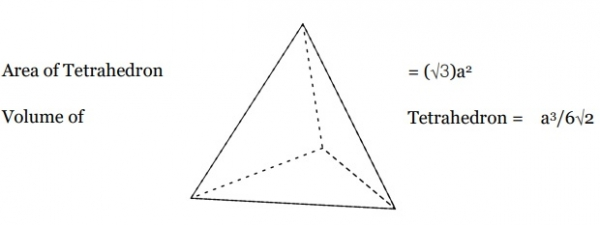# Program to calculate area and volume of a Tetrahedron

A tetrahedron is a pyramid with triangular base i.e. it has a base that is a triangle and each side has a triangle. All the three triangles converge to a point. As in the figure,Code Logic − The code to find the area and volume of tetrahedron uses the math library to find the square and square-root of a number using sqrt and pow methods. For calculating the area we take a floating point and the value of the expression “((sqrt(3)*a*a))” is given to it. Another variable get the value of volume of the tetrahedron that is evaluated by using the expression, “(a*a*a/(6*(sqrt(2))))”.

## Example

#include <stdio.h>
#include <math.h>
int main() {
int a = 5;
float area, volume;
printf("Program to find area and volume of Tetrahedron");
printf("The side of Tetrahedron is %d ", a);
area = (sqrt(3)*(a * a));
printf("The area of Tetrahedron is %f ", area);
volume = (pow(a, 3) / (6 * sqrt(2)));
printf("The volume of Tetrahedron is %f ", volume);
return 0;
}

## Output

Program to find area and volume of Tetrahedron
The side of Tetrahedron is 5
The area of Tetrahedron is 43.301270
The volume of Tetrahedron is 14.731391